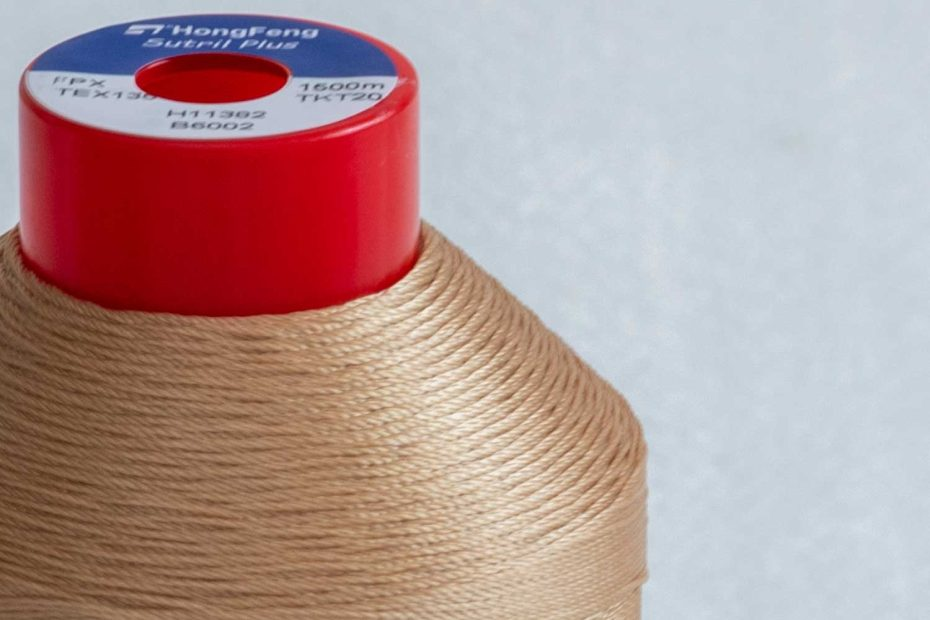# Tex Size for Industrial Sewing Thread

Threads used for industrial sewing machines (industrial sewing thread) are generally categorized differently than for home sewing. Notice the only familiar notation is “Tex”. “Tex” has been adopted by the International Organization for Standardization (IOS) as the measurement unit for sewing thread density and as such is probably going to increase in popularity. It’s in use, especially in Canada and Europe, and it’s not unusual to see threads marked in “tex” units even in the USA.

## What is Sewing Thread Tex Weight?

`Tex Size: Weight in grams of 1000 meters of thread. If 1,000 meters weighs 25 grams, it is a tex 25. Larger tex numbers are heavier threads.`

It’s common to see threads labeled with a “Tex” Number. But what does that number mean? Tex, or Tex Weight, is a standard way of measuring thread weight that’s deceptively easy to understand: the higher Tex, the thicker the thread.

A thread’s Tex is equal to the weight in grams of 1000 meters of that thread, so a heavier thread has a higher Tex. For example, if 1000 meters of a thread weighs 30 grams, it is Tex 30. Typical light-weight sewing threads range from Tex 8-25, medium-weight sewing threads are Tex 25-40, and heavy-weight sewing threads are Tex 40 and up.

The finer the thread (thinner, lighter), the lower the Tex number.

• Fine Tex Threads . . . . . . Tex 9 to Tex 19 (thin, embroidery, special)
• Medium Tex Threads . . . Tex 20 to Tex 69 (regular)
• Heavy Tex Threads . . . . . Tex 70 and higher (heavy duty, upholstery)

## Regular size

• For a sewing thread marked in weight, it would be a weight of 40. For something labeled in tex, it would be somewhere around tex 25, and for threads marked in denier, it would be 225.
• A thinner thread is usually weight 50-60 and higher, tex 20 and lower, denier 180 and lower.
• And a thicker thread is usually weight 30 and lower, tex 30 and higher, denier 300 and higher.
• Threads are marked differently in Wt. (or Tex. or Den.)numbers can have the same thickness (see the example above with the Eloflex thread). The thread thickness depends on the density of the thread, and the fibers used.

## Arriving at a Ticket Number